Courses

# Fluid Kinematics Civil Engineering (CE) Notes | EduRev

## Civil Engineering (CE) : Fluid Kinematics Civil Engineering (CE) Notes | EduRev

The document Fluid Kinematics Civil Engineering (CE) Notes | EduRev is a part of the Civil Engineering (CE) Course Civil Engineering SSC JE (Technical).
All you need of Civil Engineering (CE) at this link: Civil Engineering (CE)

Chapter 4 Fluid Kinematics

Steady & unsteady flow If the fluid and flow characteristics (such as density, velocity,  pressure etc.) at a point do not change with time, the flow is said to be steady flow. If the fluid and flow variables at a point may change with time, the flow will be unsteady. 

Uniform and Non-uniform flow If the velocity vector at all points in the flow is same at any instant of time, the flow is uniform flow. If the velocity vector varies from point to point at any instant of time, the flow will be non-uniform laminar flow generally occess at low velocities while turbulent flow occurs at high velocities. 

Laminar and Turbulent flow In laminar flow, the particles moves in layers sliding smoothly over the adjacent layers while in turbulent flow particles have the random and erratic movement, intermixing in the adjacent layers. 

Streamline 

A streamline is an imaginary line drawn in a flow field such that a tangent drawn at any point on this time represents the direction of velocity vector at that point. 

There is no velocity component normal to stream lines. 

Pathline A pathline is a curve traced by a single fluid particle during its motion. The path can cross each other. 

Streakline When a dye is injected in a liquid or smoke in a gas so as to trace the subsequent motion of liquid particles passing a fixed point, the path followed by the dye or smoke is called the streakline. 

In a steady flow streamline, pathline & streak lines are same. 

Stream tube Stream lines are drawn through a closed curve, they form a boundary surface across which fluid cannot penetrate. 

Stream-line equation in 3- D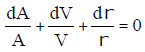Fluid Acceleration

Tangential acceleration (as ) = V/t (local acceleration) +V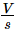(convective acceleration)

Normal acceleration (an) = Vn/2 (local acceleration) +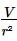(convective acceleration)

 Type of flow ConvectiveAcceleration TemporalAcceleration Steady & uniform 0 0 Steady & non-uniform Exists 0 Unsteady & uniform 0 Exists Unsteady & non-uniform Exists Exists

Acceleration in three dimensional-flow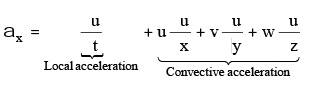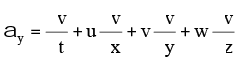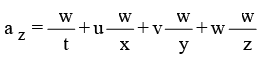Above equations are valid for steady & unsteady flows uniform & non uniform flow.  Continuity Equations For incompressible and steady 3 dimensional flow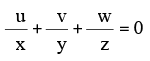For compressible and 3 dimensional unsteady flow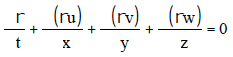For compressible one dimensional flow

r1 A1 V1 = r2 A2 V2

One dimensional differential form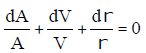For incompressible one dimensional flow A1V1 = A2V2

Circulation Circulation is defined as the summation of the product of velocity along the element of curve and element length ds.
G = òv cos qds 

• Vorticity
Vorticity is circulation per unit area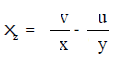Vorticity at a point, x =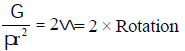xZ =circulation/area

•  Irrotational and Rotational flow 
• In irrotational flow, the vorticity is zero, at all points in the flow region while for rotational flow, vorticity is non–zero. 
• Flow outside the boundary layer has irrotational characteristic while that within the boundary layer is rotational characteristic. 
• Rotational component in x – y plane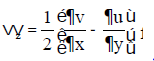W = 1/2 N Hence, w = 0

For irrotational flow in 3 – D Ñ ´ V = 0

• Velocity potential ( f)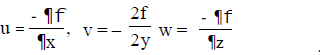•  Flow always occurs in the direction of decreasing potential. 
• If fexists and satisfies continuity equation, then it represents the possible irrotational flow.. 
• If fsatisfied Laplace equation then it is the case of steady incompressible and irrotational flow.. 
• Laplace equation is given by 22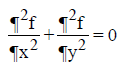• Streamline function ( y )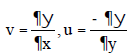•  If y exists, then it satisfies continuity equation which can be rotational or irrotational. 
• If stream function satisfies Laplace equation then it is case of irrotational flow. 
• Flow net: fand y lines intersect orthogonal to each other..
Offer running on EduRev: Apply code STAYHOME200 to get INR 200 off on our premium plan EduRev Infinity!

## Civil Engineering SSC JE (Technical)

113 docs|50 tests

,

,

,

,

,

,

,

,

,

,

,

,

,

,

,

,

,

,

,

,

,

;